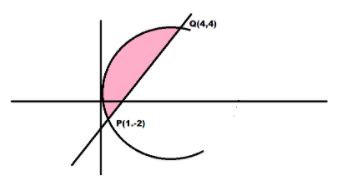QuestionAnswers

# Let $A = \left\{ {\left( {x,{\text{ }}y} \right):{y^2} \leqslant 4x,{\text{ }}y - 2x \geqslant - 4} \right\}$. Then the area (in square units) of the region A is (A) 8 (B) 9 (C) 10 (D) 11

Hint: Here first we will consider the equations of given curves and then draw their diagram and find the point of intersection of two curves and then find the area of the shaded region using the intersection points as the lower and upper limit of integration.

The given curves are:-
${y^2} = 4x$ …………………..(1)
$y - 2x = - 4$……………………………(2)
The given curves can be drawn as:-Now we will find the intersection points of the given curves.
Evaluating the value of x from equation 2 we get:-
$2x = y + 4 \\ \Rightarrow x = \dfrac{{y + 4}}{2} \\$
Putting this value in equation 1 we get:-
${y^2} = 4\left( {\dfrac{{y + 4}}{2}} \right)$
Simplifying it further we get:-
$\Rightarrow {y^2} = 2\left( {y + 4} \right) \\ \Rightarrow {y^2} - 2y - 8 = 0 \\$
For a standard quadratic equation $a{x^2} + bx + c = 0$ the quadratic formula for roots is given by:-
$x = \dfrac{{ - b \pm \sqrt {{b^2} - 4ac} }}{{2a}}$
Applying this formula for above quadratic equation we get:-
$y = \dfrac{{ - \left( { - 2} \right) \pm \sqrt {{{\left( { - 2} \right)}^2} - 4\left( 1 \right)\left( { - 8} \right)} }}{{2\left( 1 \right)}}$
Simplifying it further we get:-
$y = \dfrac{{2 \pm \sqrt {4 + 32} }}{2} \\ \Rightarrow y = \dfrac{{2 \pm \sqrt {36} }}{2} \\ \Rightarrow y = \dfrac{{2 \pm 6}}{2} \\$
Evaluating the value of y we get:-
$\Rightarrow y = \dfrac{{2 + 6}}{2};y = \dfrac{{2 - 6}}{2} \\ \Rightarrow y = \dfrac{8}{2};y = \dfrac{{ - 4}}{2} \\ \Rightarrow y = 4;y = - 2 \\$
Now putting these values in equation 1 and evaluating the respective values of x we get:-
When $y = 4$
${\left( 4 \right)^2} = 4x$
Evaluating for x we get:-
$16 = 4x \\ \Rightarrow x = \dfrac{{16}}{4} \\ \Rightarrow x = 4 \\$
When $y = - 2$
${\left( { - 2} \right)^2} = 4x$
Evaluating for x we get:-
$4 = 4x \\ \Rightarrow x = \dfrac{4}{4} \\ \Rightarrow x = 1 \\$
Hence we got the intersection points.
Let the intersection points of the two graphs be P and Q such that:
$P = \left( {1, - 2} \right)$ and $Q\left( {4,4} \right)$
Now we will evaluate the area under the curve formed by the intersection of two curves.
Hence,
${\text{Area under the curve}} = {\text{area under the line with equation }}(y - 2x = - 4) - {\text{area under the parabola with equation }}({y^2} = 4x)$
Therefore, evaluating the area with respect to y we get:-
${\text{Area under the curve}} = \int_{ - 2}^4 {\left( {\dfrac{{y + 4}}{2}} \right) - \left( {\dfrac{{{y^2}}}{4}} \right)dy}$
Now taking the LCM and solving it further we get:-
${\text{Area under the curve}} = \int_{ - 2}^4 {\left( {\dfrac{{2\left( {y + 4} \right) - {y^2}}}{4}} \right)dy} \\ {\text{ }} = \int_{ - 2}^4 {\left( {\dfrac{{2y + 8 - {y^2}}}{4}} \right)dy} \\$
Now distributing the integral we get:-
${\text{Area under the curve}} = \int_{ - 2}^4 {\left( {\dfrac{{2y}}{4}} \right)dy} + \int_{ - 2}^4 {\left( {\dfrac{8}{4}} \right)dy} - \int_{ - 2}^4 {\left( {\dfrac{{{y^2}}}{4}} \right)dy}$
Simplifying it further we get:-
${\text{Area under the curve}} = \dfrac{1}{2}\int_{ - 2}^4 {ydy} + 2\int_{ - 2}^4 {dy} - \dfrac{1}{4}\int_{ - 2}^4 {{y^2}dy}$
Now using the following formula of integration:-
$\int {{x^n}dx = \dfrac{{{x^{n + 1}}}}{{n + 1}}} + C$
We get:-
${\text{Area under the curve}} = \dfrac{1}{2}\left[ {\dfrac{{{y^2}}}{2}} \right]_{ - 2}^4 + 2\left[ y \right]_{ - 2}^4 - \dfrac{1}{4}\left[ {\dfrac{{{y^3}}}{3}} \right]_{ - 2}^4$
Simplifying it further we get:-
${\text{Area under the curve}} = \dfrac{1}{4}\left[ {{y^2}} \right]_{ - 2}^4 + 2\left[ y \right]_{ - 2}^4 - \dfrac{1}{{12}}\left[ {{y^3}} \right]_{ - 2}^4$
Now evaluating the limits we get:-
${\text{Area under the curve}} = \dfrac{1}{4}\left[ {{{\left( 4 \right)}^2} - {{\left( { - 2} \right)}^2}} \right] + 2\left[ {4 - \left( { - 2} \right)} \right] - \dfrac{1}{{12}}\left[ {{{\left( 4 \right)}^3} - {{\left( { - 2} \right)}^3}} \right]$
Simplifying it further we get:-
${\text{Area under the curve}} = \dfrac{1}{4}\left[ {16 - 4} \right] + 2\left[ {4 + 2} \right] - \dfrac{1}{{12}}\left[ {64 + 8} \right] \\ {\text{ }} = \dfrac{1}{4}\left[ {12} \right] + 2\left[ 6 \right] - \dfrac{1}{{12}}\left[ {72} \right] \\ {\text{ }} = 3 + 12 - 6 \\ {\text{ }} = 15 - 6 \\ {\text{ }} = 9{\text {sq units}} \\$
Hence the required area is 9 sq units

So, the correct answer is “Option B”.

Note: Students might make mistakes while evaluating the limits so the correct value of the limits should be should and substituted in order to get the correct answer.
Also, students should note that the general formula for integration is:
$\int {{x^n}dx = \dfrac{{{x^{n + 1}}}}{{n + 1}}} + C$